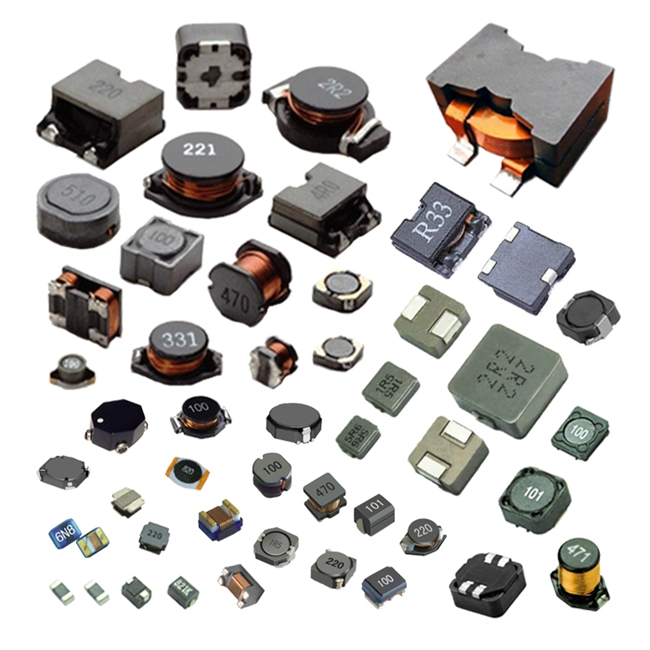# What are the main parameters of the inductor

##### author: MagTop
30/06/2022The basic parameters of the inductor are inductance, quality factor, inherent capacitance, stability, the amount of current passing through and the frequency of use.
(1) Nominal inductance: the size of the inductance marked on the inductor. Indicates the inherent characteristics of the coil itself, which mainly depends on the number of turns, structure and winding method of the coil. It has nothing to do with the size of the current. It reflects the ability of the inductive coil to store magnetic field energy, and also reflects the ability of the inductor to generate induced electromotive force when changing current. Unit for Henry (H).
(2) Allowable error: The larger allowable deviation range of the actual inductance of the inductance relative to the nominal value is called the allowable error.
(3) Inductive reactance XL: The size of the inductive coil's hindering effect on AC current is called inductive reactance XL, and the unit is ohm. Its relationship with inductance L and AC frequency f is XL=2πfL.
(4) Quality factor Q: a physical quantity representing the quality of the coil, Q is the ratio of the inductive reactance XL to its equivalent resistance, that is: Q=XL/R. The higher the Q value of the coil, the smaller the loop loss. The Q value of the coil is related to the DC resistance of the wire, the dielectric loss of the skeleton, the loss caused by the shield or iron core, and the influence of the high-frequency skin effect. The Q value of the coil is usually tens to one hundred.
(5) Rated current: The rated current refers to the working current that can ensure the normal operation of the circuit.
(6) Nominal electricity
(7) Distributed capacitance (parasitic capacitance)